# VBA LEFT Function

Various functions are common in both Excel as well as VBA. Of all the many text functions, VBA LEFT is one of the inbuilt functions that many users use to fetch only a part of the String (from the left side of the String) or value given by the user.

If you have already worked with the LEFT function in the Excel worksheet, you can easily work with the VBA LEFT function because both are exactly the same. This tutorial will discover the definition, syntax, arguments, and various examples explaining the real use of the VBA LEFT function.

## What is VBA LEFT Function?

“The VBA LEFT Function is used to extract the length of characters (or a substring) only from the left side of the string or the value given by the user.”

Using this function in a VBA code returns a substring from the input string from the starting part. In other words, the VBA LEFT function only fetches the substring from the leftmost side of the input string. For instance, you have an input string, “Hello World”, you can fetch the first 5 characters, i.e., HELLO, using the VBA LEFT function.

This function can be used as a VBA function and a worksheet function in excel. It can be used as a part of the formula in the cell. The VBA LEFT function is listed under the text category of VBA functions. It just functions like the LEFT function in the Excel worksheet.

### Parameter

• String (required): The String argument represents the length of the string we are trying to extract.
• Length (required): This length argument represents but how many characters you want to fetch from the left side of the specified String.

### Return

The VBA LEFT Function returns a substring after extracting the leftmost string from the main string.

### Points to Remember

1. The VBA Left function returns a string-based output for a given string.
2. IF the specified string argument is Null, this function will return Null as the output.
3. As the name suggests, the VBA Left function extracts only the leftmost characters from the string.
4. When the LEFT function is combined with the InStr function, we can find the space in VBA; by this, a user can easily differentiate the word in the specified string.

## Examples

### LEFT Function Example 1: Fetch the first name from the string “Reema Panda”.

VBA LEFT is one of the inbuilt functions that many users use to fetch only a part of the String (from the left side of the String) or value given by the user.

Below given are the steps to write a VBA macro to fetch the first name from the specified string using the VBA Left function:

Step 1: Open the VBA developer tab. Go to Excel worksheet, from the ribbon tab click on developer window -> visual basic editor or directly click on the shortcut keywords Alt +F11.

Step 2: The VB Editor window will be displayed. The next step is to create a module. From the ribbon tab click on Insert-> Click on Module.Step 3: You will notice, VBA will insert a new module window. Start the program by introduce your macro name followed by the declaration of the variable.

Refer to the below given macro code:

Step 4: Next, we will incorporate the LEFT function. In the arguments, we will pass the String, and in the length argument, we will pass 5 since we want to extract the first 5 characters from the leftmost part of the String.

Store the fetch value in our variable and later return the variable using MsgBox.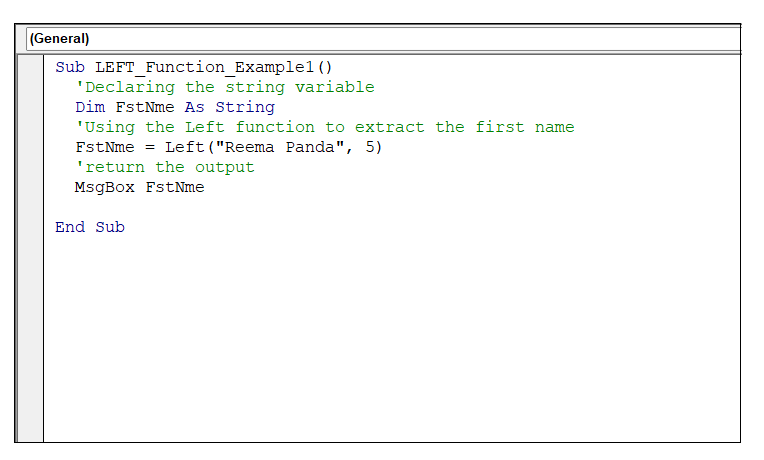Output

Run your macro either by clicking Run or by pressing the F5 key. As a result, you will notice VBA will throw a MsgBox displaying the first 5 characters from the leftmost side of your string.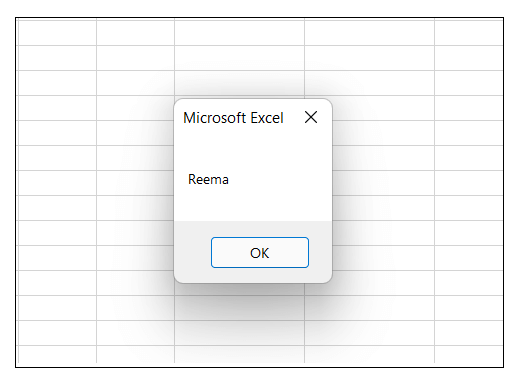The macro has extracted the first 5 characters from the string “Reema Panda.” So the output will display first name, i.e., “Reema”.

### LEFT Function Example 2: Fetch the first name from employee table.

Many often, you are asked to extract the first name from the Excel table. VBA LEFT function is a great advantage to solve these types of cases.

Below given in an employee table. Let’s see another example where we will be using the VBA Left Function for extracting the first name of the employee from the employee table.Below given are the steps to write a VBA macro to fetch the first name from the specified employee table using the VBA Left function:

Step 1: Open the VBA developer tab. Go to Excel worksheet, from the ribbon tab click on developer window -> visual basic editor or directly click on the shortcut keywords Alt +F11.

Step 2: The VB Editor window will be displayed. The next step is to create a module. From the ribbon tab click on Insert-> Click on Module.Step 3: You will notice, VBA will insert a new module window. Start the program by introduce your macro name followed by the declaration of the variable.

Refer to the below given macro code:

Step 4: Since we have to apply the function for a range of cells in Excel so we will incorporate the FOR loop. Start loop and assign variable FstNme the result of the VBA left function.

Refer to the below code:

Step 5: Just to confirm that your program has run successfully, add a MsgBox displaying a message.

Refer to the below code:

Output

Run your macro either by clicking Run or by pressing the F5 key. As a result, you will notice VBA will throw a MsgBox and will fetch the first names of each Employee from the Employee table.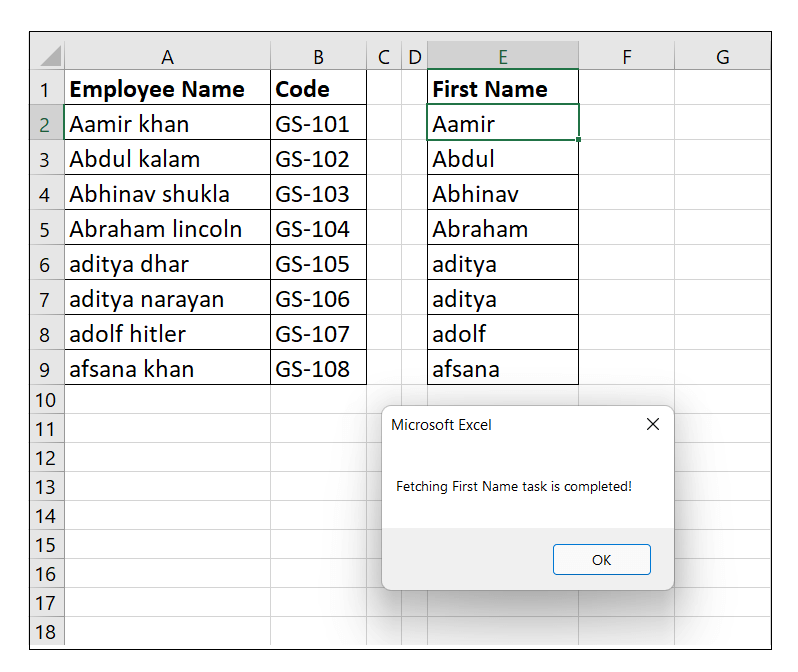### LEFT Function Example 3: Fetch the salary for employees in thousands.

The VBA LEFT Function is useful to extract the salary of employees in thousands.

Below given is the employee table where we are given the employee name and along with it the respective salaries of each employee.Below given are the steps to write a VBA macro to display only the salary in thousands of each employee:

Step 1: Open the VBA developer tab. Go to Excel worksheet, from the ribbon tab click on developer window -> visual basic editor or directly click on the shortcut keywords Alt +F11.

Step 2: The VB Editor window will be displayed. The next step is to create a module. From the ribbon tab click on Insert-> Click on Module.Step 3: You will notice, VBA will insert a new module window. Start the program by introduce your macro name followed by the declaration of the variable.

Refer to the below given macro code:

Step 4: Since we have to apply the function for a range of cells in Excel so we will incorporate the FOR loop. Start loop and assign variable sal the result of the VBA left function.

Refer to the below code:

Step 5: Just to confirm that your program has run successfully, add a MsgBox displaying a message.

Refer to the below code: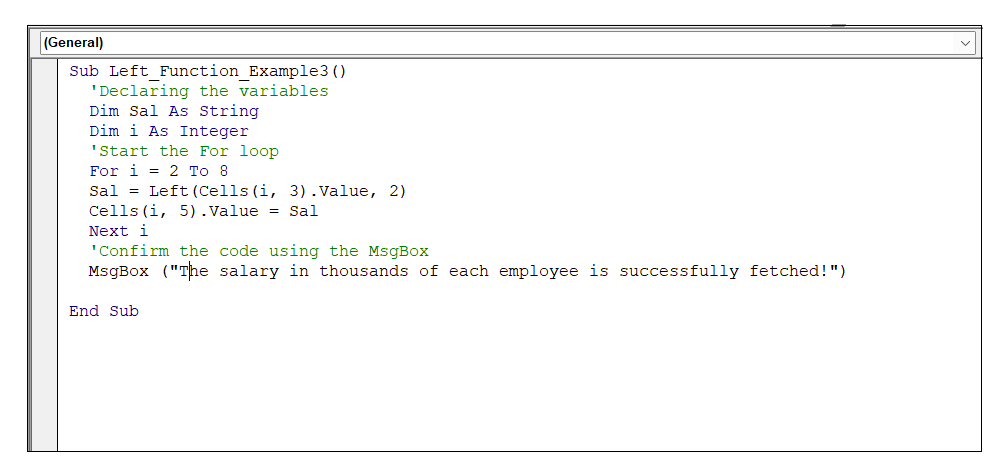Output

Run your macro either by clicking Run or by pressing the F5 key. As a result, you will notice VBA will throw a MsgBox displaying the message and it will fetch the salary in thousands for all its employees.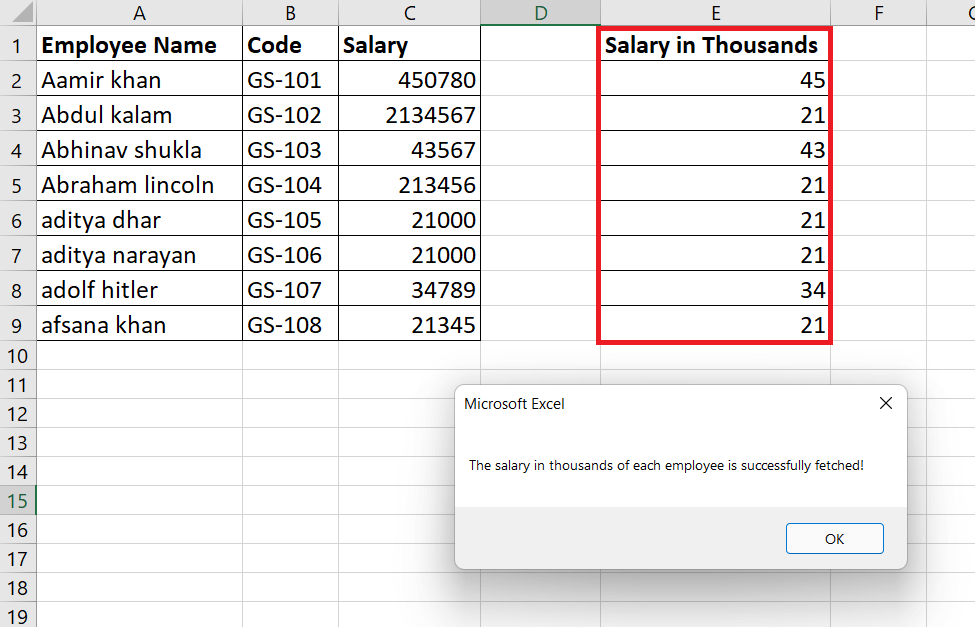Next TopicVBA TRIM Function

Previous articleVBA Data Types
Next articleCircular reference in Excel